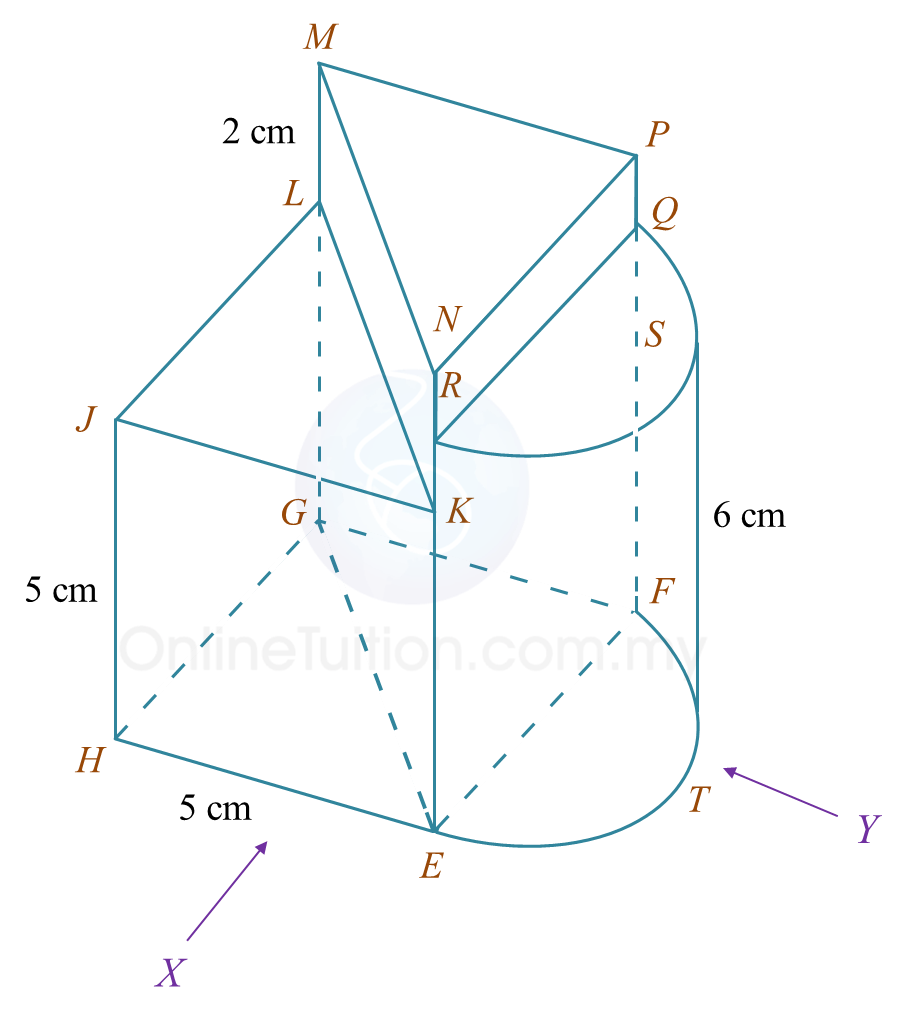10.3 SPM Practice (Long Questions)

Question 6 (12 marks):
You are not allowed to use graph paper to answer this question.

(a) Diagram 6.1 shows two solid right prisms joined at the vertical plane EGLK. The planes JKL and MNP are the uniform cross sections of the prism HEGLJK and prism EFGMNP respectively. The base EFGH is a rectangle which lies on a horizontal plane. Edges HJ and EK are vertical.Diagram 6.1

Draw, to full scale, the plan of the composite solid.

(b)
Another solid half cylinder with a diameter of 4 cm is joined to the prism in Diagram 9.1 at the vertical plane EFQR. The composite solid is as shown in Diagram 6.2. The base HETFG lies on a horizontal plane.Diagram 6.2

Draw to full scale,
(i) the elevation of the composite solid on a vertical plane parallel to HE as viewed from X.
(ii) the elevation of the composite solid on a vertical plane parallel to EF as viewed from Y.

Solution:
(a)(b)(i)(b)(ii)